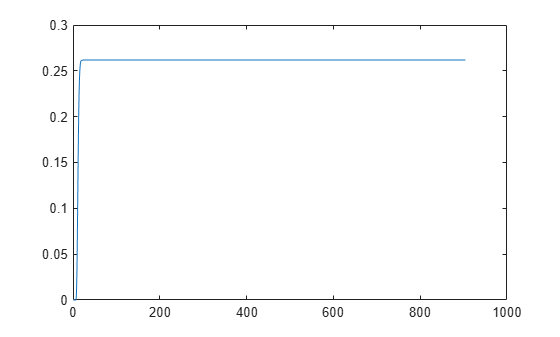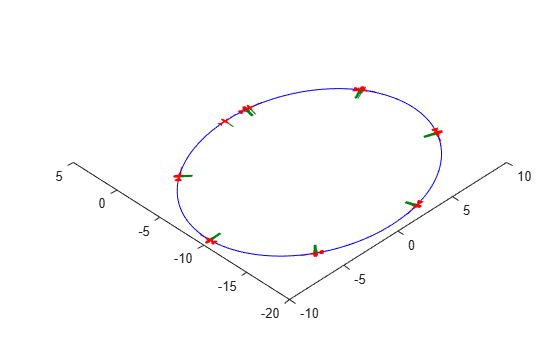# fixedwing

Guidance model for fixed-wing UAVs

## Description

A `fixedwing` object represents a reduced-order guidance model for an unmanned aerial vehicle (UAV). The model approximates the behavior of a closed-loop system consisting of an autopilot controller and a fixed-wing kinematic model for 3-D motion.

For multirotor UAVs, see `multirotor`.

## Creation

`model = fixedwing` creates a fixed-wing motion model with `double` precision values for inputs, outputs, and configuration parameters of the guidance model.

`model = fixedwing(DataType)` specifies the data type precision (`DataType` property) for the inputs, outputs, and configurations parameters of the guidance model.

## Properties

expand all

Name of the UAV, used to differentiate it from other models in the workspace, specified as a string scalar.

Example: `"myUAV1"`

Data Types: `string`

UAV controller configuration, specified as a structure of parameters. Specify these parameters to tune the internal control behavior of the UAV. Specify the proportional (P) and derivative (D) gains for the dynamic model and other UAV parameters. The structure for fixed-wing UAVs contains these fields with defaults listed:

• `'PDRoll'` - `[3402.97 116.67]`

• `'PHeight'` - `3.9`

• `'PFlightPathAngle'` - `39`

• `'PAirspeed'` - `0.39`

• `'FlightPathAngleLimits'` - `[-pi/2 pi/2]` (`[min max]` angle in radians)

Example: ```struct('PDRoll',[3402.97,116.67],'PHeight',3.9,'PFlightPathAngle',39,'PAirSpeed',0.39,'FlightPathAngleLimits',[-pi/2 pi/2])```

Data Types: `struct`

UAV guidance model type, specified as `'FixedWingGuidance'`.

Input and output numeric data types, specified as either `'double'` or `'single'`. Choose the data type based on possible software or hardware limitations.

## Object Functions

 `control` Control commands for UAV `derivative` Time derivative of UAV states `environment` Environmental inputs for UAV `state` UAV state vector

## Examples

collapse all

This example shows how to use the `fixedwing` guidance model to simulate the change in state of a UAV due to a command input.

Create the fixed-wing guidance model.

`model = fixedwing;`

Set the air speed of the vehicle by modifying the structure from the `state` function.

```s = state(model); s(4) = 5; % 5 m/s```

Specify a control command, `u`, that maintains the air speed and gives a roll angle of `pi/12`.

```u = control(model); u.RollAngle = pi/12; u.AirSpeed = 5;```

Create a default environment without wind.

`e = environment(model);`

Compute the time derivative of the state given the current state, control command, and environment.

`sdot = derivative(model,s,u,e);`

Simulate the UAV state using `ode45` integration. The `y` field outputs the fixed-wing UAV states based on this simulation.

```simOut = ode45(@(~,x)derivative(model,x,u,e), [0 50], s); size(simOut.y)```
```ans = 1×2 8 904 ```

Plot the change in roll angle based on the simulation output. The roll angle is the 7th row of the `simOut.y` output.

`plot(simOut.y(7,:))`You can also plot the fixed-wing trajectory using `plotTransforms`. Create the translation and rotation vectors from the simulated state. Downsample (every 30th element) and transpose the `simOut` elements, and convert the Euler angles to quaternions. Specify the mesh as the `fixedwing.stl` file and the positive Z-direction as `"down"`. The displayed view shows the UAV making a constant turn based on the constant roll angle.

```downsample = 1:30:size(simOut.y,2); translations = simOut.y(1:3,downsample)'; % xyz-position rotations = eul2quat([simOut.y(5,downsample)',simOut.y(6,downsample)',simOut.y(7,downsample)']); % ZYX Euler plotTransforms(translations,rotations,... 'MeshFilePath','fixedwing.stl','InertialZDirection',"down") hold on plot3(simOut.y(1,:),-simOut.y(2,:),simOut.y(3,:),'--b') % full path xlim([-10.0 10.0]) ylim([-20.0 5.0]) zlim([-0.5 4.00]) view([-45 90]) hold off```expand all

 Randal W. Beard and Timothy W. McLain. "Chapter 9." Small Unmanned Aircraft Theory and Practice, NJ: Princeton University Press, 2012.

## SupportGet trial now